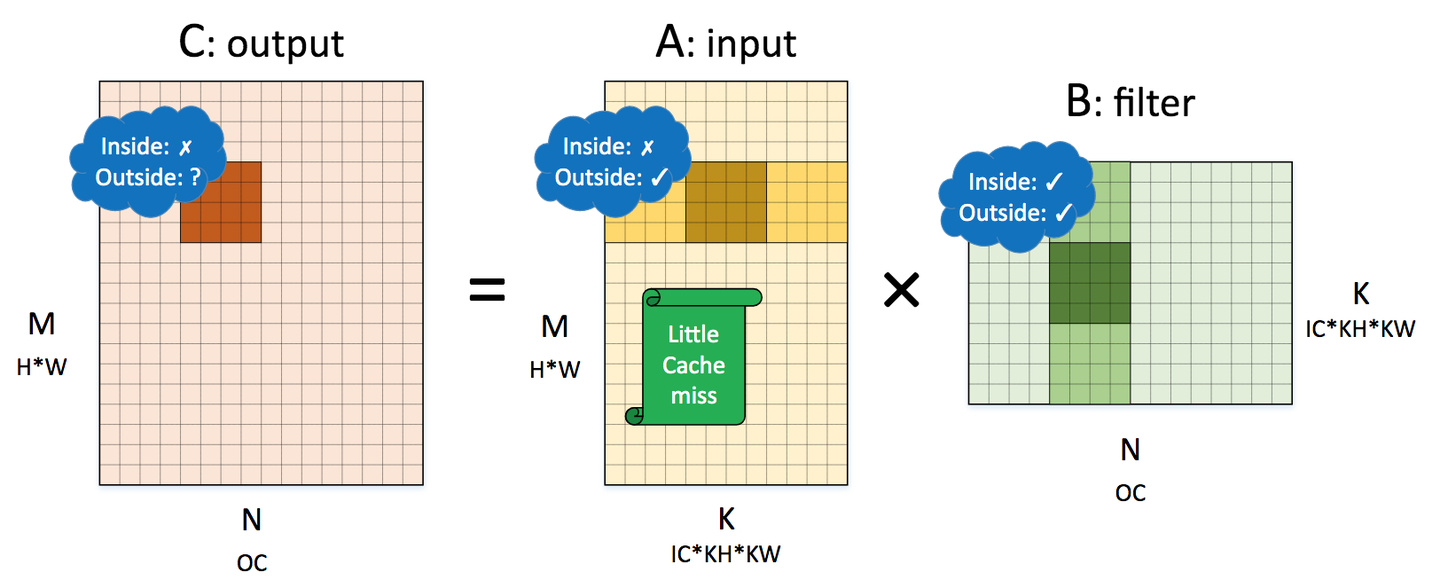GEMM 主题文章写了两版，这是第一版，第二版参见我的博客

## 通用矩阵乘优化

### 基本概念

for (int m = 0; m < M; m++) {
for (int n = 0; n < N; n++) {
C[m][n] = 0;
for (int k = 0; k < K; k++) {
C[m][n] += A[m][k] * B[k][n];
}
}
}

How to optimize gemm 介绍了如何采用各种优化方法，将最基础的计算改进了约七倍（如图二）。其基本方法是将输出划分为若干个 4×4子块，以提高对输入数据的重用。同时大量使用寄存器，减少访存；向量化访存和计算；消除指针计算；重新组织内存以地址连续等。详细的可以参考原文。

### 计算拆分展示

for (int m = 0; m < M; m++) {
for (int n = 0; n < N; n += 4) {
C[m][n + 0] = 0;
C[m][n + 1] = 0;
C[m][n + 2] = 0;
C[m][n + 3] = 0;
for (int k = 0; k < K; k++) {
C[m][n + 0] += A[m][k] * B[k][n + 0];
C[m][n + 1] += A[m][k] * B[k][n + 1];
C[m][n + 2] += A[m][k] * B[k][n + 2];
C[m][n + 3] += A[m][k] * B[k][n + 3];
}
}
}

for (int m = 0; m < M; m += 4) {
for (int n = 0; n < N; n += 4) {
C[m + 0][n + 0..3] = 0;
C[m + 1][n + 0..3] = 0;
C[m + 2][n + 0..3] = 0;
C[m + 3][n + 0..3] = 0;
for (int k = 0; k < K; k++) {
C[m + 0][n + 0..3] += A[m + 0][k] * B[k][n + 0..3];
C[m + 1][n + 0..3] += A[m + 1][k] * B[k][n + 0..3];
C[m + 2][n + 0..3] += A[m + 2][k] * B[k][n + 0..3];
C[m + 3][n + 0..3] += A[m + 3][k] * B[k][n + 0..3];
}
}
}

for (int m = 0; m < M; m += 4) {
for (int n = 0; n < N; n += 4) {
C[m + 0..3][n + 0..3] = 0;
C[m + 0..3][n + 0..3] = 0;
C[m + 0..3][n + 0..3] = 0;
C[m + 0..3][n + 0..3] = 0;
for (int k = 0; k < K; k += 4) {
C[m + 0..3][n + 0..3] += A[m + 0..3][k + 0] * B[k + 0][n + 0..3];
C[m + 0..3][n + 0..3] += A[m + 0..3][k + 1] * B[k + 1][n + 0..3];
C[m + 0..3][n + 0..3] += A[m + 0..3][k + 2] * B[k + 2][n + 0..3];
C[m + 0..3][n + 0..3] += A[m + 0..3][k + 3] * B[k + 3][n + 0..3];
}
}
}

### 处理内存布局

for (int mo = 0; mo < M; mo += 8) {
for (int no = 0; no < N; no += 8) {
for (int mi = 0; mi < 2;mi ++) {
for (int ni = 0; ni < 2; ni++) {
int m = mo + mi * 4;
int n = no + ni * 4;
C[m + 0..3][n + 0..3] = 0;
C[m + 0..3][n + 0..3] = 0;
C[m + 0..3][n + 0..3] = 0;
C[m + 0..3][n + 0..3] = 0;
for (int k = 0; k < K; k += 4) {
C[m + 0..3][n + 0..3] += A[m + 0..3][k + 0] * B[k + 0][n + 0..3];
C[m + 0..3][n + 0..3] += A[m + 0..3][k + 1] * B[k + 1][n + 0..3];
C[m + 0..3][n + 0..3] += A[m + 0..3][k + 2] * B[k + 2][n + 0..3];
C[m + 0..3][n + 0..3] += A[m + 0..3][k + 3] * B[k + 3][n + 0..3];
}
}
}
}
}

## QNNPACK 的矩阵乘优化

QNNPACK (Quantized Neural Network PACKage) 是 Facebook 开源的专门用于量化神经网络的计算加速库。QNNPACK 和 NNPACK (Neural Network PACKage) 的作者都是 Marat Dukhan 。到目前为止，QNNPACK 仍然是已公开的，用于移动端（手机）的，性能最优的量化神经网络加速库。

QNNPACK 开源时附带了一份技术报告性质的博客。本节将结合上节的内容简要地从博客原作中抽取一些关于 GEMM 的内容。

### 量化神经网络### 计算划分与削减维度

QNNPACK 实现了 4×8 的和 8×8 两种计算核（micro kernel），分别用于支持 armv7 和 arm64 指令集的处理器。这两种计算核在原理上区别不大，后者主要利用了更多的寄存器和双发射（Dual Issue）以提高计算的并行度。

## 卷积与矩阵乘

### im2col 计算方法

im2col 是计算机视觉领域中将图片的不同通道（channel）转换成矩阵的列（column）的计算过程。Caffe 在计算卷积时，首先用 im2col 将输入的三维数据转换成二维矩阵，使得卷积计算可表示成两个二维矩阵相乘，从而充分利用已经优化好的 GEMM 库来为各个平台加速卷积计算。

im2col 计算卷积使用 GEMM 的代价是额外的内存开销，输入会使用额外的 × 倍内存。当卷积核尺寸是 1×1 时，由于不需要重排输入，GEMM 可以直接在原始输入上运行，并且不需要使用额外的内存。